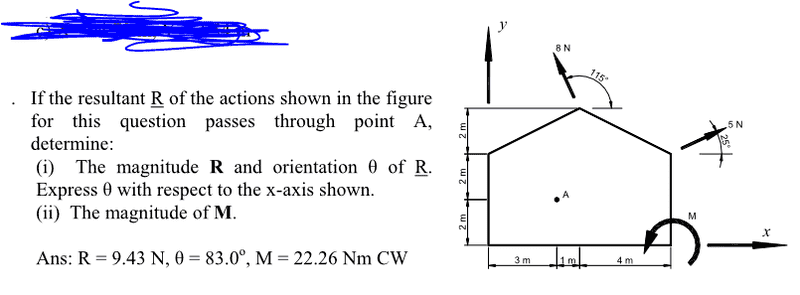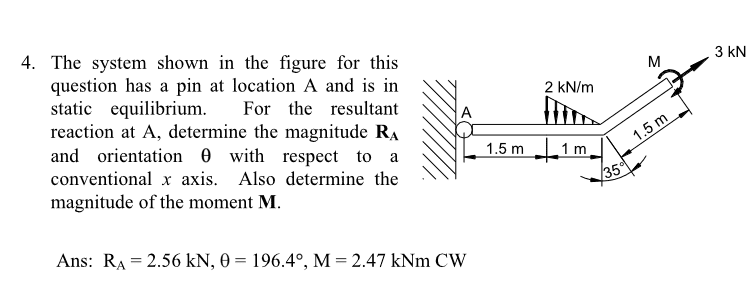# Moments and the line of action of the resultant force

nonyabus

## Homework Statement## Homework Equations

ƩMoments = Moment + Fx*d + Fy*d

R = (Fx)i + (Fy)j

## The Attempt at a Solution

I'm only interested in part (ii)

I calculated the components of the resultant force and they are

|R| = 1.15(i) + 9.36(j)

I know that the x-intercept of line of action is sum of moments divided by R(j) and y-intercept is sum of moments divided by R(i). I'm given a point A where the line of action goes through but I'm not quite sure how to use that bit of information to find M.

Any help is appreciated.

nonyabus
I have figured it out.

Since we know where the line of action acts, we must find the magnitude of the moment (M) that makes it act at that location. If we take moments about point A, then (M) is equivalent to the actions of the two forces about point A.

So I broke the two forces into their components, force × perpendicular distance... yadda yadda and I got the correct answer!

nonyabusI don't know what this one is asking for, really... is it the resultant force on point A? Or the reaction force?

What I tried doing is represent the 2 kNm as 0.8N acting 2.5m away from A and normally calculate the moment by splitting the forces into components.

I'm not getting the right answer... here is my attempt:
R(i) = 3 cos 35

R(j) = -0.8 + 3 sin 35

|R|= 2.62 kN??

nonyabus
Clues on the 2nd question, anyone?

nonyabus
O.K. seems like I was wrong about the 2kN/m force. It can NOT be represented by a point load of 0.8N with distance 2.5m.

The magnitude of the force is the area of the triangle and where it acts is somewhere called the "centroid" (of a right triangle) and acts at two third's of its base.

http://www.ele.uri.edu/~daly/106/06/project/centroid/centroid.html